﻿ 基于方向可调滤波和概率霍夫变换的车道线检测方法 Lane Line Detection Method Based on Steerable Filters and Probability Hough Transform

Open Journal of Transportation Technologies
Vol. 08  No. 02 ( 2019 ), Article ID: 29188 , 8 pages
10.12677/OJTT.2019.82014

Lane Line Detection Method Based on Steerable Filters and Probability Hough Transform

Mian Zhang, Shang Gao, Wanda Chi

Shandong University of Science and Technology, Qingdao Shandong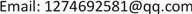Received: Feb. 16th, 2019; accepted: Mar. 4th, 2019; published: Mar. 11th, 2019ABSTRACT

In the lane line detection, images obtained by the vehicle-mounted camera contain a lot of noise and interference. To improve the speed and accuracy of the lane line detection, a lane line detection method is proposed in this paper based on steerable filters and probability Hough transform. The mean and variance of the direction angle of the lane line are obtained by means of statistics to determine the direction of steerable filters and polar angle range of probability Hough transform, which can effectively inhibit interference, reduce the search range, and improve the detection speed. In terms of the experimental results, the proposed method in this paper can effectively eliminate non-lane interference and accurately detect the lane line, and therefore, is characterized by a high robustness.

Keywords:Lane Line Detection, Steerable Filters, Probability Hough Transform, Polar Angle Constraint1. 引言

2. 车道线检测方法

2.1. 总体框架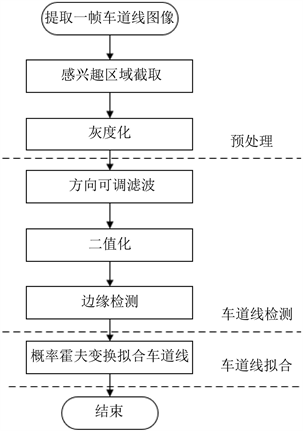Figure 1. Framework of lane line detection

1) 感兴趣区域截取。车道线主要位于图像的下半部分，为兼顾准确度与实时性，本文提取图像底部的2/3区域为感兴趣区域。

2) 灰度化。人眼对绿色敏感度最高，对蓝色敏感度最低，对彩色图像中的R、G、B三个分量加权平均得到灰度图像，其灰度值计算如公式(1)：

$Y=0.3\text{R}+0.59\text{G}+0.11\text{B}$ (1)

3) 方向可调滤波。根据统计的左右车道线方向角均值和方差  ，确定方向可调滤波器的输入角并进行边缘增强。方向可调滤波器为二维高斯函数的一阶导数的线性组合。

4) 图像二值化。利用Otsu  阈值对图像进行二值化。

5) 边缘检测。利用Canny算子进行图像边缘检测。

6) 概率霍夫变换拟合车道线。根据统计的左右车道线方向角均值和方差，对左右车道线极角范围进行限定，并利用概率霍夫变换对车道线进行拟合。

2.2. 基于方向可调滤波的车道线检测

$G\left(x,y\right)=\mathrm{exp}\left(-\left({x}^{2}+{y}^{2}\right)\right)$ (2)

${G}_{1}^{0˚}=\frac{\partial }{\partial x}\mathrm{exp}\left(-\left({x}^{2}+{y}^{2}\right)\right)=-2x\mathrm{exp}\left(-\left({x}^{2}+{y}^{2}\right)\right)$ (3)

${G}_{1}^{90˚}=\frac{\partial }{\partial y}\mathrm{exp}\left(-\left({x}^{2}+{y}^{2}\right)\right)=-2y\mathrm{exp}\left(-\left({x}^{2}+{y}^{2}\right)\right)$ (4)

${G}_{1}^{\theta }={G}_{1}^{0˚}\mathrm{cos}\theta +{G}_{1}^{90˚}\mathrm{sin}\theta$ (5)

${I}^{\theta }=f*{G}_{1}^{\theta }=f*{G}_{1}^{0˚}\mathrm{cos}\theta +{G}_{1}^{90˚}\mathrm{sin}\theta =\mathrm{cos}\theta +\mathrm{sin}\theta$ (6)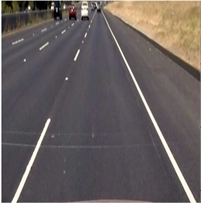(a)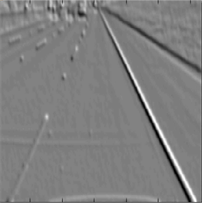(b)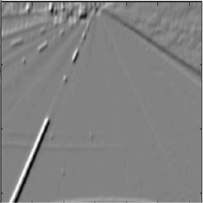(c)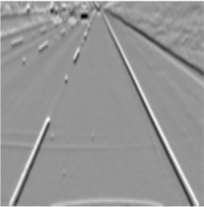(d)(e)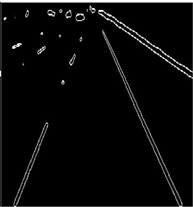(f)

Figure 2. Process result of lane line detection. (a) Region of interest, (b) Image ${I}^{\theta 1}$ after filters processing, (c) Image ${I}^{\theta 2}$ after filters processing, (d) Image I after merged, (e) Binarization, (f) Edge detection

2.3. 基于概率霍夫变换的车道线拟合

1) 建立并初始化一个累加器 $A\left(\rho ,\theta \right)$ ，将车道线检测获取的边缘图像前景点放入待选边缘点集S；

2) 集合S若为空则算法结束；否则从集合S中随机取一点 $\left(x,y\right)$ 投射到参数空间，并从S中去掉该点，然后在 ${\theta }_{l}$${\theta }_{r}$ 范围内计算相应的 $\rho$ 值，并更新对应的累加器 $A\left(\rho ,\theta \right)$

3) 对 $A\left(\rho ,\theta \right)$ 与预定的阈值比较，若大于阈值且满足最小线段长度，则执行步骤4)，否则回到步骤2)；

4) 取出该直线，完成一条直线的拟合。

5) 删除S中位于直线上的所有点，并将累加器清零，再继续执行步骤2)。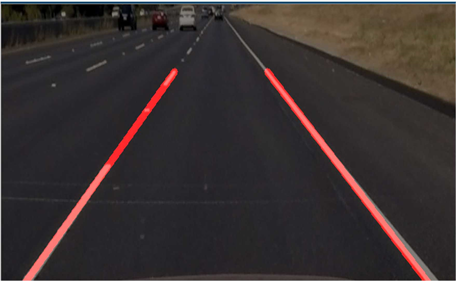Figure 3. Result of lane line fitting detection

3. 实验结果与对比

3.1. 车道线检测实验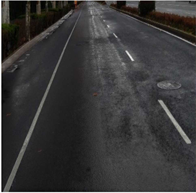(a)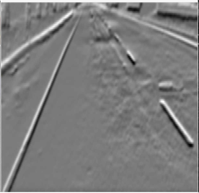(b)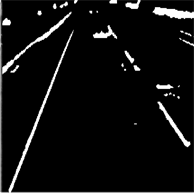(c)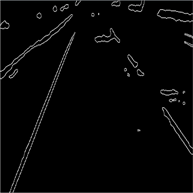(d)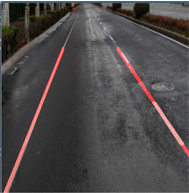(e)

Figure 4. Result of rainy day lane line detection. (a) Region of interest, (b) Steerable filters, (c) Binarization, (d) Canny edge detection, (e) Result of lane line detection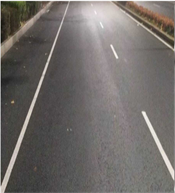(a)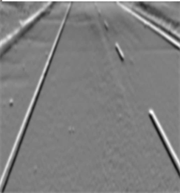(b)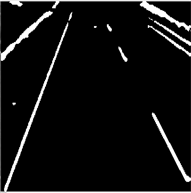(c)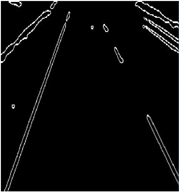(d)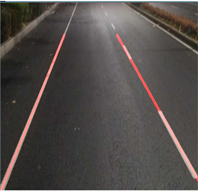(e)

Figure 5. Result of night strong light lane line detection. (a) Region of interest, (b) Steerable filters, (c) Binarization, (d) Canny edge detection, (e) Result of lane line detection

3.2. 与其它方法的对比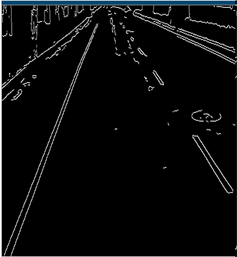(a)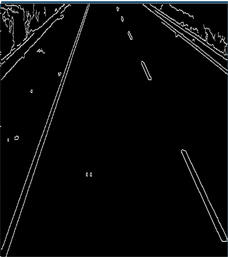(b)

Figure 6. Canny edge detection result of literature  algorithm. (a) Process result of rainy day image, (b) Process result of night image

4. 结论

Lane Line Detection Method Based on Steerable Filters and Probability Hough Transform[J]. 交通技术, 2019, 08(02): 112-119. https://doi.org/10.12677/OJTT.2019.82014

1. 1. 凌诗韵, 马乐, 黄楚然, 等. 基于Hough变换的城市环境车道识别优化算法研究[J]. 机械设计与制造工程, 2017(12): 54-57.

2. 2. Yim, Y.U. and Oh, S.Y. (2003) Three-Feature Based Automatic Lane Detection Algorithm (TFALDA) for Autonomous Driving. IEEE Transactions on Intelligent Transportation Systems, 4, 219-225.

3. 3. Li, Q., Zheng, N.N. and Cheng, H. (2004) Spring Robot: A Prototype Autonomous Vehicle and Its Algorithms for Lane Detection. IEEE Transactions on Intelligent Transportation Systems, 5, 300-308. https://doi.org/10.1109/TITS.2004.838220

4. 4. Li, X., Zhang, W.G. and Bian, X.D. (2004) Research on Detection of Lane Based on Machine Vision. Journal of Southeast University (English Edition), 20, 176-180.

5. 5. Thomas, B., Cristobal, C., Johann, E., et al. (2003) Image Processing and Behavior Planning for Intelligent Vehicles. IEEE Transactions on Industrial Electronics, 50, 62-75. https://doi.org/10.1109/TIE.2002.807650

6. 6. 胡徐怡, 王超, 厉丹. 基于改进Sobel算子的边缘检测算法研究[J]. 福建电脑, 2018, 14(4): 13-14.

7. 7. Freeman, W.T. and Adelson, E.H. (1991) The Design and Use of Steerable Filters. IEEE Transactions on Pattern Analysis and Machine Intelligence, 13, 891-906. https://doi.org/10.1109/34.93808

8. 8. 张荣, 王勇, 杨榕. TM图像中车道目标识别方法的研究[J]. 遥感学报, 2005, 9(2): 220-224.

9. 9. 孙丰荣, 刘积仁. 快速霍夫变换算法[J]. 计算机学报, 2001, 24(10): 1102-1109.

10. 10. 邹乐强. 最小二乘法原理及其简单应用[J]. 科技信息, 2010(23): 282-283.

11. 11. 李大新. 基于机器视觉的车道线检测识别与车道偏离预警算研究[D]: [硕士学位论文]. 山东: 山东大学, 2012.

12. 12. 曾接贤. 霍夫变换与最小二乘法相结合的直线拟合[J]. 南昌航空工业学院学报: 自然科学版, 2003, 17(4): 9-13.

13. 13. 胡彬, 赵春霞. 基于概率霍夫变换的快速车道线检测方法[J]. 微电子学与计算机, 2011, 28(10): 177-180.

14. 14. Jung, C.R. and Kelber, C.R. (2004) A Robust Linear-Parabolic Model for Lane Following. Proceedings of the XVII Brazilian Symposium on Computer Graphics and Image Processing Curitiba, 10, 72-79.

15. 15. Zhang, H.L., Lu, B.C. and Yu, Y. (2008) The Method of Ostu Used in the Video Detection of Vehicles. International Conference on Intelligent Computation Technology and Automation (ICICTA), Hunan, 20-22 October 2008, 547-551. https://doi.org/10.1109/ICICTA.2008.219

16. 16. Galamhos, C., Matas, J. and Kittler, J. (1999) Progressive Probabilistic Bough Transform for Line Detection. Proceedings of Computer Vision and Pattern Recognition Fort Collins, Fort Collins, CO, 23-25 June 1999, 23-35.

17. 17. 李亚娣, 黄海波, 李相鹏, 等. 基于Canny算子和Hough变换的夜间车道线检测[J]. 科学技术与工程, 2016, 16(31): 234-237, 242.